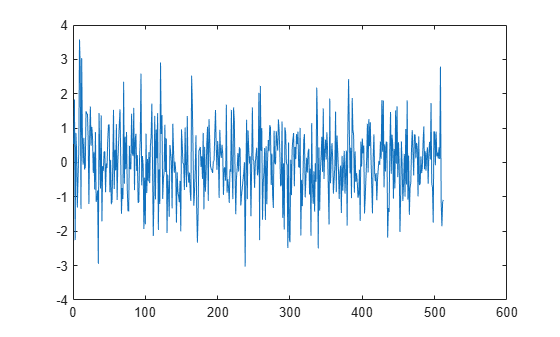## Syntax

``out = read(asyncBuff)``
``out = read(asyncBuff,NumRows)``
``out = read(asyncBuff,NumRows,Overlap)``
``[out,nUnderrun] = read(___)``

## Description

````out = read(asyncBuff)` returns all unread samples from the buffer, `asyncBuff`.```

example

````out = read(asyncBuff,NumRows)` returns `NumRows` samples from each channel (column) of the buffer.```
````out = read(asyncBuff,NumRows,Overlap)` returns `NumRows` samples from each channel and overlaps previously read samples by `Overlap`.```
````[out,nUnderrun] = read(___)` also returns the number of rows zero-padded if underrun occurred, using any of the previous arguments.```

## Examples

collapse all

The `dsp.AsyncBuffer` System object™ supports reading variable frame sizes from the buffer.

Create a `dsp.AsyncBuffer` System object. The input is white Gaussian noise with a mean of 0, a standard deviation of 1, and a frame size of 512 samples. Write the input to the buffer using the `write` method.

```asyncBuff = dsp.AsyncBuffer; input = randn(512,1); write(asyncBuff,input); plot(input) hold on```Store the data that is read from the buffer in `outTotal`.

Plot the input signal and data that is read from the buffer in the same plot. Read data from the buffer until all samples are read. In each iteration of the loop, `randi` determines the number of samples to read. Therefore, the signal is read in as a variable-size signal. The `prevIndex` variable keeps track of the previous index value that contains the data.

```outTotal = zeros(size(input)); prevIndex = 0; while asyncBuff.NumUnreadSamples ~= 0 numToRead = randi([1,64]); out = read(asyncBuff,numToRead); outTotal(prevIndex+1:prevIndex+numToRead) = out; prevIndex = prevIndex+numToRead; end plot(outTotal,'r') hold off```Verify that the input data and the data read from the buffer (excluding the underrun samples, if any) are the same. The cumulative number of overrun and underrun samples in the buffer is determined by the `info` function.

`S = info(asyncBuff)`
```S = struct with fields: CumulativeOverrun: 0 CumulativeUnderrun: 28 ```

The `CumulativeUnderrun` field shows the number of samples underrun per channel. Underrun occurs if you attempt to read more samples than available.

## Input Arguments

collapse all

Async buffer, specified as a `dsp.AsyncBuffer` System object.

Number of samples read from each channel (column) of the buffer, specified as a positive integer. If the requested number of samples is greater than the number of unread samples, the output is zero-padded.

The function returns `NumRows` samples from each channel and overlaps previously read samples by `Overlap`. The total number of samples read is `NumRows` × NumChann, where NumChann is the number of channels in the buffer. The total number of new samples read is (`NumRows``Overlap`) × NumChann. If the overlap portion contains samples that are overwritten, and are therefore not contiguously written, the output is zero-padded.

## Output Arguments

collapse all

Data read from the buffer, returned as an array of `NumRows` × NumChann samples. If `Overlap` is specified, the function returns (`NumRows``Overlap`) × NumChann samples. If the requested number of samples is greater than the number of unread samples, the output is zero-padded.

Data Types: `double`
Complex Number Support: Yes

Number of samples zero-padded in each channel (column) if underrun occurred. Underrun occurs if you attempt to read more samples than available. Samples that are zero-padded in overlapped portions are not counted as underrun.

Data Types: `int32`

## Version History

Introduced in R2017a